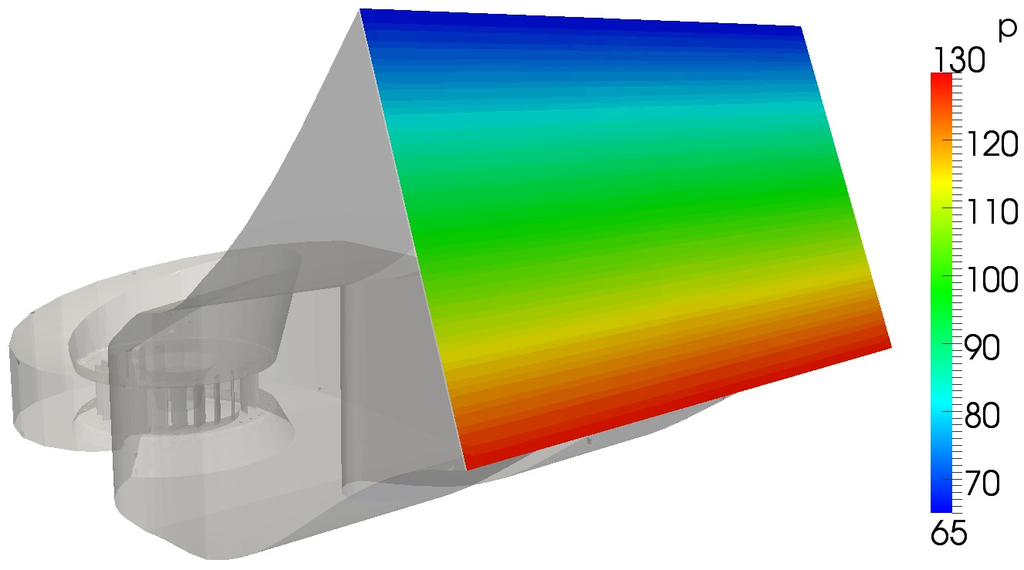## Hydrostatic Total Pressure Boundary Condition

When computing within gravity the hydrostatic pressure is employed. Hydrostatic total pressure is simple total pressure, but gravity gradient is applied. Total pressure at the inlet patch center is equal pInletCenter, the vertical deviation is employed due to the gravity (reading constant/g).

p:

``` boundaryField
{
".*_inlet"
{
type            hydrostaticTotalPressure;
pInletCenter    97.5;
value           \$internalField;
phi             phi;
}
}
```

U:

``` boundaryField
{
".*_inlet"
{
type            pressureInletVelocity;
value           \$internalField;
}
}
```Figure: When computing within gravity. Distribution of total pressure at the inlet can be prescribed using hydrostaticTotalPressure boundary condition with reference value pInletCenter.